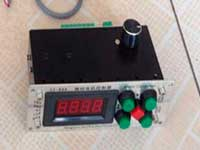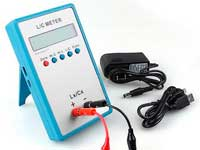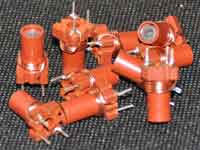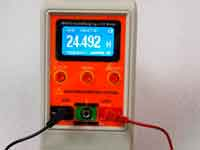The self-capacitance of a single-layer air core solenoid - 4.4 out of 5 based on 8 votes
Rating 4.38 (8 Votes)

Is there, I ask, can there be, a more interesting study than that of alternating currents

Nicola Tesla

The self-capacitance of a single-layer air core solenoid

At high frequencies, the inductor would be correctly represented as a helical single-wire transmission line. As is known, such a structure is defined as the small inductances and capacitances distributed along the line length. When the length of this line is equal to λ / 4 (λ - wavelength of the electromagnetic wave in the coil), the line is in resonance mode and the frequency corresponding to this wavelength is called the self-resonant frequency of the coil. Coils rarely operate at frequencies close to the self-resonance or above. However, if this happens, such as in RF choke, the calculation of the coil is preferable to carry out in an RF electromagnetic simulators.Typically, the design of the coil is selected such that it operates at a frequency much lower self-resonant.  In such case, the coil with sufficient accuracy can be represented as an equivalent circuit of the ideal lumped elements. In this representation, the coil is connected in parallel its own parasitic capacitance. With the self-inductance of the coil, it forms parallel LC circuit, the resonant frequency of which is the self-resonant frequency of the coil. This capacitance depends on the material of the coil-former, shape of winding, availability of screen and core. To simplify the problem, we define to a first approximation, how a form of winding influences on a value of the parasitic capacitance.

In the evolution of the radio repeatedly attempts were made to pick up the empirical formula for the calculation of self-capacitance.

• The first time the question of self-capacitance of the coil inductance was raised by J.C. Hubbard in 1917. S.Butterworth, all known innovator and designer of frequency filtering circuits (remember Butterworth filters) in 1926 proposed a formula for the calculation, but it had serious limitations and could not count short coil. (What is meant by the term "short coil", read the article dedicated to the q-factor)
• There was invented a method of determining the self-capacitance of the coil by indirect measurement. The resonant frequencies of the LC circuit with two different external capacitors are measured and then from the measurement results is calculated the self-capacitance and self-inductance of the coil.However, it requires measurement equipment. Moreover, by this method, we can determine the self-capacitance of the finishing made inductor but we desired to estimate this parameter at the stage of design.

The formula proposed by the engineer AJ Palermo in 1934 in the paper named "Distributed Capacity of Single-Layer Coils":Cs = 2 π D / arccosh (p / d) 
• Сs - self-capacitance (pF);
• D - diameter of winding (cm);
• p - winding pitch (cm);
• d - wire diameter (cm);

This formula quite common can be found in a variety of references, but as shown by subsequent experience - the formula is not right! Palermo represents the coil as an electrostatic structure in which the self-capacitance is obtained as the sumof series capacitance between adjacent turns. Logical conclusion - by increasing gap between adjacent turns we decrease this total capacitance.

Historically the solenoid began to be studied as a device with a static magnetic field at the DC. Because of it the distributed self-capacitance also was defined as electrostatic capacitance. But it was a main mistake. Despite the apparent obviousness and clarity, this approach is wrong. As I noted at the outset, the RF coil is the distributed structure around which there is an electromagnetic field, which is the single entity in which the vectors of the electric and magnetic fields are always perpendicular and the electrical and magnetic components of the field cannot exist independently of each other.

Since the magnetic field lines are mainly located along the axis of the coil, the electric vector of the wave is located perpendicular to the axis, and the components of the electric field parallel to the axis (between turns) are almost negligible.

Formula G. Grandi, published in article "Stray Capacitances of Single-Layer Solenoid Air-Core Inductors - Grandi, Kazimierczuk, Massarini and Reggiani 1999":

 Cs = ε0 π2 D / ((N-1) ln{ ( p/d ) + √[ ( p/d )2 - 1 ] }) 
• N - number of turns;
• ε0 - dielectric constant;
• D - coil diameter (cm);
• p - winding pitch (cm);
• d - wire diameter (cm).

It was the attempt of modern reanimation electrostatic model of the coil, that as shown in the case of formula Palermo, far from the reality. It is a vivid example, when "paper theory" is not rightly verified by experiment and nevertheless is accepted as the truth. From the formula we conclude, if a coil has the more turns, then the less a parasitic capacitance, what is absolute conflicts with practice.

In 1947 radio engineer R. G. Medhurst - an employee of research laboratory of the "General Electric Co.Ltd." has published a number of works related to experimental research of inductors. A careful measurement of the parasitic capacity of a large number of coils, Medhurst came to the conclusion that the self-capacitance of the coil is only weakly dependent on the pitch of winding and model Palermo does not work. Also, Medhurst found a dependence of the self-capacitance from the form factor of a coil that is the ratio of the length of the winding to its diameter l/D). On the basis of these measurements Medhurst derived his semi-empirical formula:

 Cs = H * D 
• D- coil diameter (cm),
• H - coefficient characterizing the form factor of the coil that was presented in the form of a table:
l/DHl/DHl/DH
50 5.8 5 0.81 0.7 0.47
40 4.6 4.5 0.77 0.6 0.48
30 3.4 4 0.72 0.5 0.50
25 2.9 3.5 0.67 0.45 0.52
20 2.36 3 0.61 0.4 0.54
15 1.86 2.5 0.56 0.35 0.57
10 1.32 2 0.50 0.3 0.60
9 1.22 1.5 0.47 0.25 0.64
8 1.12 1 0.46 0.2 0.70
7 1.01 0.9 0.46 0.15 0.79
6 0.92 0.8 0.46 0.1 0.96

However, the more famous the most simple formula for the estimation of the self-capacitance of the one-layer air core coil, which was proposed by Medhurst:

 Cs = 0.46 * D 
• Cs - self-capacitance [pF];
• D - coil diameter [cm];

The self-capacitance of a single-layer air core coil in pF is numerically approximately equal to the radius of the winding in cm, but we need to remember that this is true when 0.5 < l/D < 2, otherwise we have to take the factor from the table. However, R. G. Medhurst did not explain his experimental results and the question "What is the self-capacitance?" remained open.

A complete modern work that dedicated self-capacitance of the coil is the article "The self-resonance and self-capacitance of solenoid coils" Dr. David W Knight 2013" (G3YNH) [ref. 1 ⬇]
A few quotes from this work:There is even a school of thought that says that the self-capacitance is due to the capacitance between adjacent turns; and although that is partly true for multi-layer coils and flat spirals, the hypothesis turns out to be a hopeless predictor of the reactance of single-layer solenoids.

The solution, of course, lies in recognizing that the coil is a transmission-line; except that the line in question turns out to be a rather complicated one.

A trivial investigation involving a Grid-Dip Oscillator and a set of engineer's callipers will confirm that the various resonances exhibited by a disconnected coil are associated primarily with the total conductor length. It is therefore extraordinary that the self-capacitance of single-layer coils is still routinely attributed to the static capacitance that is presumed exist between adjacent turns.

The calculation of the self-capacitance of single-layer coils in the program Coil32 is based on the Dr. David W Knight essay without considering the influence of the coil-former. It is understood that the inductor operates at a frequency much lower than the self-resonance frequency and it is an air core coil. Approximately can be considered that the presence of the coil-former increases the self-capacitance compared with an estimated up to 15..30%, former with grooves under the wire increases the capacitance up to 40%, the impregnation and enveloping coils with varnish or compound increases the self-capacitance of up to 50% and above.

Summing up, you can come to the following conclusions:The concept of the self-capacitance is directly related to the self-resonant frequency of the coil. Hence, when considering the physical processes in a single-layer coil at frequencies close to the frequency of its self-resonance it is necessary to abandon the model of lumped elements as baseless and to consider the inductor as a transmission line. In the "straight transmission line", such parameters as a capacitance and inductance per length depends only on its geometry. In the spiral line, due to the influence of turns on each other, these parameters are also a function of frequency. This fact leads to important consequences:

• The resonance frequencies of this line though severely are depending on the total length of the conductor which is wound the coil, but not multiples of each other.
• The self-capacitance of the coil depends on the frequency at which it is determined. Ignore the effect of frequency and talk about the calculation of the capacitance of the coil makes sense only when the coil operates at frequencies not exceeding 60-70% from the frequency of its self-resonance. (In fairness it should be noted that this applies to the calculation of inductance!)
• At frequencies much lower than the frequency of the first resonance there is possible for simply using the lumped elements model for the coil. However, it should not be forgotten that their self-capacitance, in this case, is the summary capacitance per length along the line and depends on the length of the conductor.

How the geometry of the coil affects its self-capacitance?

• The self-capacitance of a single-layer air core coil is directly proportional to its diameter.
• The optimal coil in terms of parasitic capacitance (capacitance - minimum), is a coil with l/D ≈ 1. The same coil is optimal in terms of the quality factor. This is understandable because the coil with such a geometry winding has a maximum inductance at a minimum wire length.
• As shown above, increasing gap between the turns has almost no influence on the value of the self-capacitance. Modifying the pitch of winding, first of all, we change the inductance of the coil, and not its self-capacitance. This comes from the fact that adjacent turns are interacting with each other mainly through the magnetic field, and not through an electric.

The anonymous who has read this article has a reasonable question. If the self-capacitance of the single-layer coil is not the capacitance between the adjacent turns then between what? In the classical theory of the transmission lines, for the two wires line, we can answer a similar question because the configuration of electric field lines of a TEM wave and of electrostatic field in such line are similar. In the helix single-layer coil, there are TE and TM  waves for which such coincidence doesn't exist and therefore formulation of the question "where is the condenser?" does not have a sense. Nobody looks for any virtual condensers, for example, in a round wave-guide or Sommerfeld's line. Strictly speaking, we can talk only about an input impedance of the helix wave-guide. The coil self-capacitance concept appears when we subtract from this impedance the inductive reactance that calculated for the ideal coil with the configuration of magnetic field coinciding with the field of the solenoid at the zero frequency without wave effects. In other words, the negative reactance obtaining at such subtraction can be viewed not as the physical capacitance but just as the mathematical correction for wave effects when calculating of the ideal lumped inductance (see more ref.). We call this negative reactance the self-capacitance owing to the historical reasons and also for the convenience of calculations, but it is necessary to understand that it is not corresponding to any static capacitance. We can look for virtual "floating" condensers in a helix wave-guide with the help of a surface impedance concept, but this approach won't lead us in any way to the disreputable electrostatic "inter-turn capacitance". You can say: "Hey buddy! You're wholly madman!" If the self-capacitance is not the capacitance and it is just the mathematical correction, then how is there the self-resonance in the coil? It isn't necessary for the self-resonance of the single-layer coil. Just as it isn't necessary to look for any lumped static capacitance to explain a cavity resonance effect in a hollow wave-guide (see ref.).

References:

Сomments from anonymous guests are enabled with moderation.

Coil Winding MachineUS \$187.53
The system consists of individual components including a controller, a stepping motor, a foot switch, power adapter and output shaft. This design makes it very easy for you to integrate the system into your own project.

LC Meter LC200AUS \$37.99
Measuring Capacitance Range:
0.01 pF - 100mF
Measuring Inductance Range:
0.001 uH - 100H
Measurement accuracy:1%.

RF InductorsUS \$6.43
Variable Ferrite Core Inductor coil set 3.5Turns 25-100MHZ

Auto Ranging LCR MeterUS \$63.07
Meter can measure 0.00pF to 100.00mF capacitance, 0.000uH to 100.00H inductance and 0.00? to 10.000M? resistance. Very suitable for small value capacitor and inductor measurement.